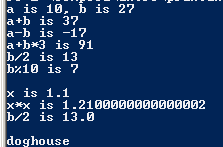# Assignemnt #17 and Math Operations

## Code

```/// Name: Raff Lisbona
/// Period: 6
/// Program Name: Math Operations
/// File Name: MathOperations.java
/// Date Finished: 9/23/2015

public class MathOperations
{
public static void main( String[] args )
{
int a, b, c, d, e, f, g;
double x, y, z;
String one, two, both;

a = 10;
b = 27;
System.out.println( "a is " + a + ", b is " + b );

c = a + b;
System.out.println( "a+b is " + c );
d = a - b;
System.out.println( "a-b is " + d );
e = a+b*3;
System.out.println( "a+b*3 is " + e );
f = b/2;
System.out.println( "b/2 is " + f );
g = b%10;
System.out.println( "b%10 is " + g );

x = 1.1;
System.out.println( "\nx is " + x );
y = x*x;
System.out.println( "x*x is " + y );
z = b/2;
System.out.println( "b/2 is " + z );
System.out.println();

one = "dog";
two = "house";
both = one + two;
System.out.println( both );
}
}
```

### Picture of the output